StatLect

# Marginal probability density function

Consider a random vector whose entries are continuous random variables, called a continuous random vector. When taken alone, one of the entries of the random vector has a univariate probability distribution that can be described by its probability density function. This is called marginal probability density function, in order to distinguish it from the joint probability density function, which instead describes the multivariate distribution of all the entries of the random vector taken together.## Definition

A more formal definition follows.

Definition Letbecontinuous random variables forming arandom vector. Then, for each, the probability density function of the random variable, denoted by, is called marginal probability density function.

Recall that the probability density functionis a functionsuch that, for any interval, we have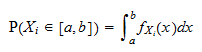whereis the probability thatwill take a value in the interval.

Instead, the joint probability density function of the vectoris a functionsuch that, for any hyper-rectangle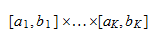we have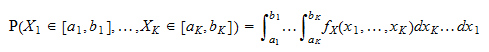whereis the probability thatwill take a value in the interval, simultaneously for all.

## How to derive it

The marginal probability density function ofis obtained from the joint probability density function as follows: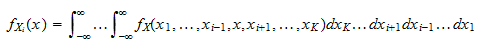In other words, the marginal probability density function ofis obtained by integrating the joint probability density function with respect to all variables except.

## Example

Letbe acontinuous random vector having joint probability density function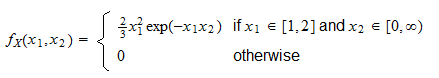The marginal probability density function ofis obtained by integrating the joint probability density function with respect to. When, then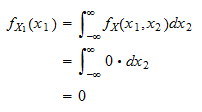When, then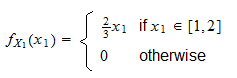Therefore, the marginal probability density function ofis## More details

Marginal probability density functions are discussed in more detail in the lecture entitled Random vectors.